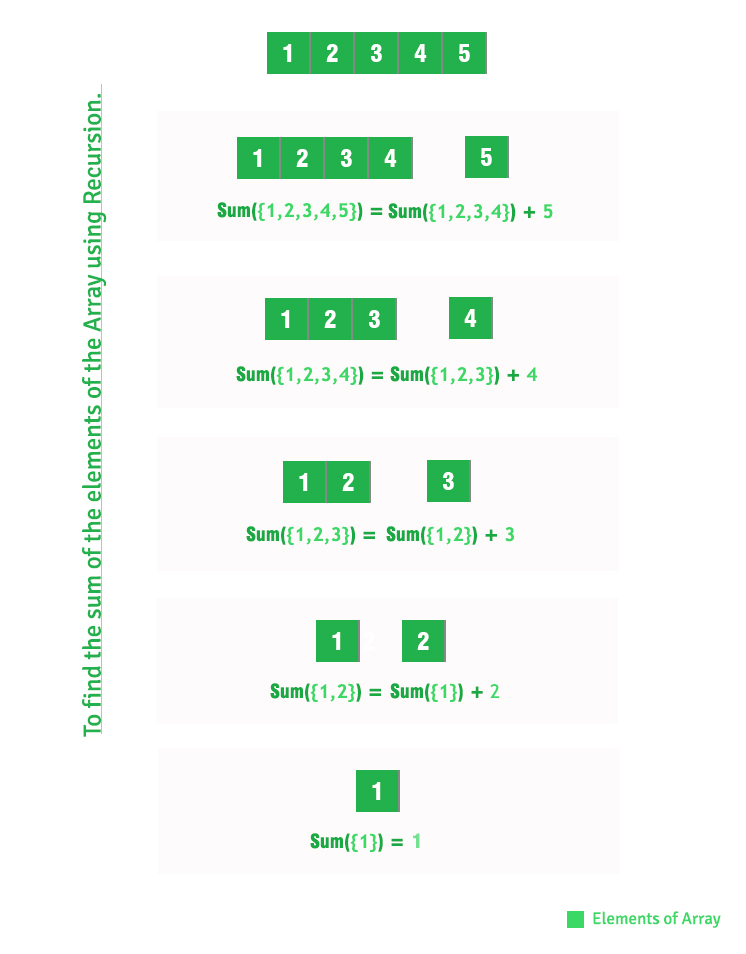Skip to content
Related Articles
Sum of array elements using recursion
• Difficulty Level : Basic
• Last Updated : 03 Aug, 2018

Given an array of integers, find sum of array elements using recursion.

Examples:

```Input : A[] = {1, 2, 3}
Output : 6
1 + 2 + 3 = 6

Input : A[] = {15, 12, 13, 10}
Output : 50
```

## Recommended: Please solve it on “PRACTICE ” first, before moving on to the solution.

We have discussed iterative solution in below post.
Sum of elements in a given array

In this post, recursive solution is discussed.

## C++

 `// C++ program to find sum of array``// elements using recursion.``#include `` ` `// Return sum of elements in A[0..N-1]``// using recursion.``int` `findSum(``int` `A[], ``int` `N)``{``    ``if` `(N <= 0)``        ``return` `0;``    ``return` `(findSum(A, N - 1) + A[N - 1]);``}`` ` `// Driver code``int` `main()``{``    ``int` `A[] = { 1, 2, 3, 4, 5 };``    ``int` `N = ``sizeof``(A) / ``sizeof``(A);``    ``printf``(``"%dn"``, findSum(A, N));``    ``return` `0;``}`

## Java

 `// Java program to find sum of array``// elements using recursion.`` ` `class` `Test {``    ``static` `int` `arr[] = { ``1``, ``2``, ``3``, ``4``, ``5` `};`` ` `    ``// Return sum of elements in A[0..N-1]``    ``// using recursion.``    ``static` `int` `findSum(``int` `A[], ``int` `N)``    ``{``        ``if` `(N <= ``0``)``            ``return` `0``;``        ``return` `(findSum(A, N - ``1``) + A[N - ``1``]);``    ``}`` ` `    ``// Driver method``    ``public` `static` `void` `main(String[] args)``    ``{``        ``System.out.println(findSum(arr, arr.length));``    ``}``}`

## Python

 `# Python program to find sum of array``# elements using recursion.`` ` ` ``# Return sum of elements in A[0..N-1]`` ``# using recursion.``  ``def` `_findSum(arr, N):``     ``if` `len``(arr)``=``=` `1``:``        ``return` `arr[``0``]``     ``else``:``        ``return` `arr[``0``]``+``_findSum(arr[``1``:], N)`` ` `# driver code``arr ``=``[]``# input values to list``arr ``=` `[``1``, ``2``, ``3``, ``4``, ``5``]``  ` `# calculating length of array``N ``=` `len``(arr)``  ` `ans ``=``_findSum(arr,N)``print` `(ans)``  ` `# This code is contributed by Khare Ishita`

## C#

 `// C# program to find sum of array``// elements using recursion.``using` `System;`` ` `class` `Test {``     ` `    ``static` `int` `[]arr = {1, 2, 3, 4, 5};`` ` `    ``// Return sum of elements in ``    ``// A[0..N-1] using recursion.``    ``static` `int` `findSum(``int` `[]A, ``int` `N)``    ``{``        ``if` `(N <= 0)``            ``return` `0;``        ``return` `(findSum(A, N - 1) + A[N - 1]);``    ``}`` ` `    ``// Driver Code``    ``public` `static` `void` `Main()``    ``{``        ``Console.Write(findSum(arr, arr.Length));``    ``}``}`` ` `// This code is contributed by Nitin Mittal.`

## PHP

 ``

Output:
```15
```

How does above recursive solution work?This article is contributed by Prakhar Agrawal. If you like GeeksforGeeks and would like to contribute, you can also write an article using contribute.geeksforgeeks.org or mail your article to contribute@geeksforgeeks.org. See your article appearing on the GeeksforGeeks main page and help other Geeks.

Please write comments if you find anything incorrect, or you want to share more information about the topic discussed above.

Attention reader! Don’t stop learning now. Get hold of all the important DSA concepts with the DSA Self Paced Course at a student-friendly price and become industry ready.  To complete your preparation from learning a language to DS Algo and many more,  please refer Complete Interview Preparation Course.

In case you wish to attend live classes with industry experts, please refer Geeks Classes Live

My Personal Notes arrow_drop_up